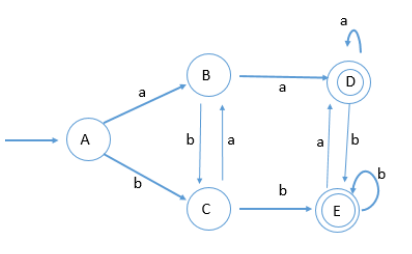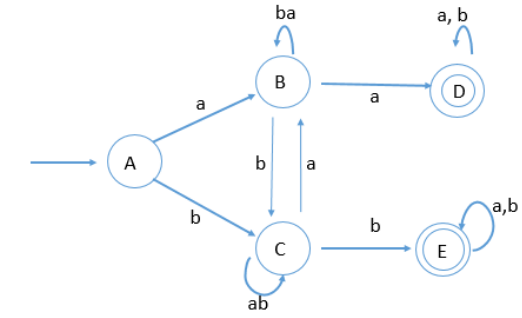# GATE | Gate IT 2007 | Question 71

Consider the regular expression R = (a + b)* (aa + bb) (a + b)*<br>

Which one of the regular expressions given below defines the same language as defined by the regular expression R?
(A) (a(ba)* + b(ab)*)(a + b)+
(B) (a(ba)* + b(ab)*)*(a + b)*
(C) (a(ba)* (a + bb) + b(ab)*(b + aa))(a + b)*
(D) (a(ba)* (a + bb) + b(ab)*(b + aa))(a + b)+

Explanation: <!–A accepts ab but given does not
B empty may be accepted, not in given –>The above DFA can be simplified by resolving the loop at states B and C and removing extra transitions at the final states which is as follows:To reach to a final state from B we can have alphabet ‘a’ to move to state D or ‘bb’ to move to state E.
Similarly, to reach to a final state from C we can have alphabet ‘b’ to move to state E or ‘aa’ to move to state D.

Hence the regular expression is:
(a(ba)*(a+bb) + b(ab)*(b+ aa))(a+b)*

a(ba)*(a+bb): to move from A to B and B to any one of the final states
b(ab)*(b+ aa): to move from A to C and C to any one of the final states

Note: We can also use Option Elimination method for such questions i.e. we can reject an option if we

find at least such string that does not validate the regular expression.
Option (A) accepts ab but given regular expression does not.
Option (B) accepts an empty string but given regular expression does not.
Option (D) does not accept aa but the given regular expression does.

This solution is contributed by Yashika Arora

Quiz of this Question

My Personal Notes arrow_drop_up
Article Tags :

Be the First to upvote.

Please write to us at contribute@geeksforgeeks.org to report any issue with the above content.